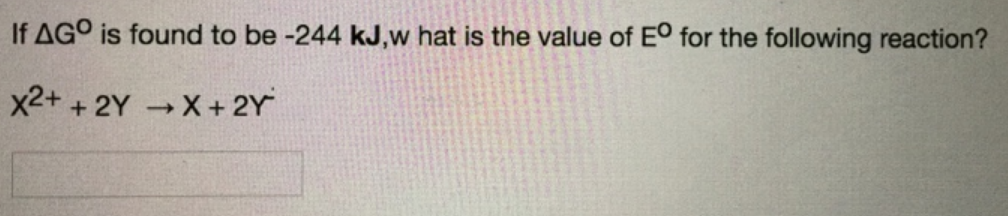# Problem: If ΔG°  is found to be -244 kJ, what is the value of E° for the following reaction?X2+ + 2Y → X + 2Y -

###### FREE Expert Solution
100% (335 ratings)###### Problem Details

If ΔG°  is found to be -244 kJ, what is the value of E° for the following reaction?

X2+ + 2Y → X + 2Y -